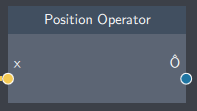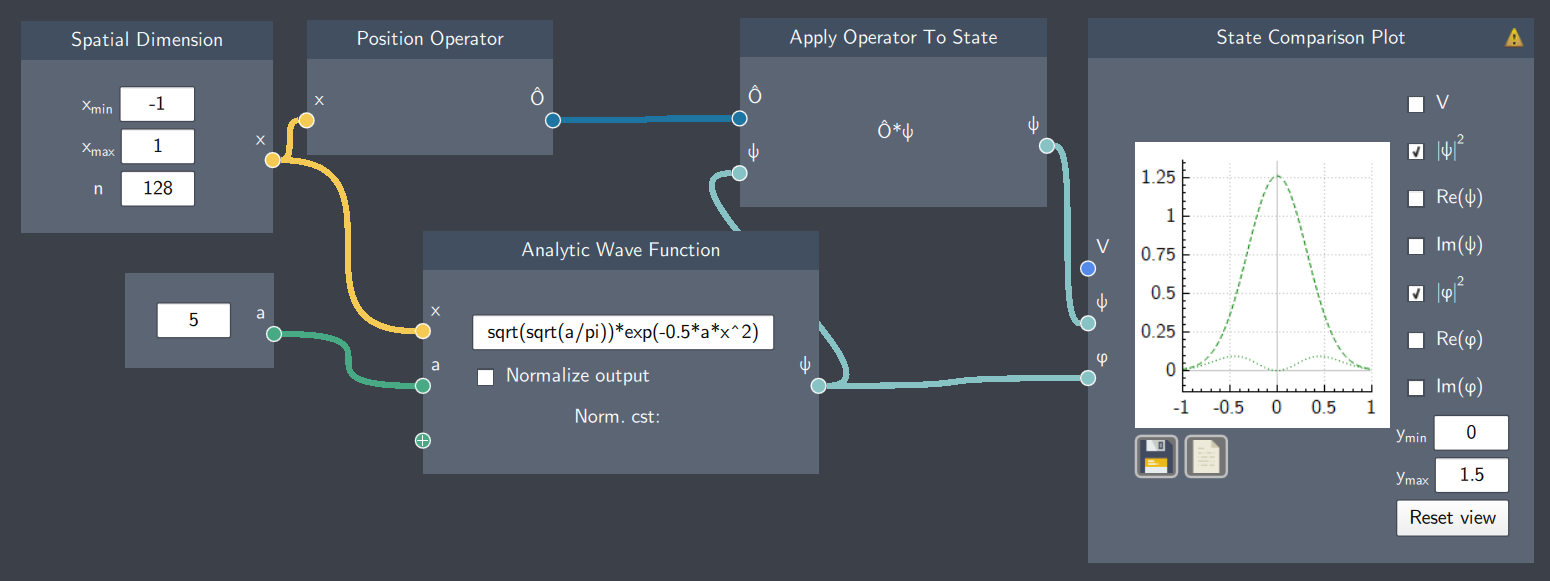# Position Operator

## Description

The Position Operator is the quantum mechanical operator which gives an instruction to multiply by $x$ (position).## Input

It has the following input:

• Spatial dimension ($x$): Inputs the spatial dimension $x$ as defined in the Spatial Dimension node.

## Content

It consists of values of $x$.

## Output

• Position Operator: The position operator $\hat{x}$ which can be applied to a state.

## Example

In the example below, the Position Operator node inputs the values of $x$ and applies it to a Gaussian function and gives a new function. The magnitudes of both the Gaussian and the resulting function after operator application are shown on the plot.